# Modelization and Simulation of a Low Cost Concentrated Photovoltaic Solar Cell: Parametric and Sensitivity Study under MATLAB

Modelization and Simulation of a Low Cost Concentrated Photovoltaic Solar Cell: Parametric and Sensitivity Study under MATLAB

Zineb AqachmarMustapha Raoufi Abdelali El Gourari Tarik Bouhal Mariame Jenhi Bekkay Hajji Abdelfettah Barhdadi

ALTES Energy, Alternative Energy Solutions, Zagora 47900, Morocco

Laboratory of Electrical Engineering and Energy System, Faculty of Sciences, University Ibn Tofail, Kenitra 14000, Morocco

National School of Applied Sciences, University Mohammed Premier, Oujda 60000, Morocco

PSES Research Team, Energy Research Center, ENS, Mohammed V University in Rabat, Rabat 10000, Morocco

Corresponding Author Email:
zineb.aqachmar@uca.ac.ma
Page:
1-6
|
DOI:
https://doi.org/10.18280/i2m.210101
24 December 2021
|
Accepted:
21 February 2022
|
Published:
28 February 2022
| Citation

OPEN ACCESS

Abstract:

The concentrated photovoltaic solar cells are the most efficient among photovoltaic technologies. This paper studies a low-cost concentrated photovoltaic solar cell made of silicon that is cheap, available, and easy to manufacture coupled to a concentrator. The novelty of this work consists on replacing the expensive triple-junction solar cell with a Silicon cell. A modelization of the electronic equivalent circuit of the low cost concentrated photovoltaic solar cell is given. The equations describing the current, voltage, and power of the CPV cell are presented. Subsequently, the computation program of the implemented MATLAB code is shown. Afterward, the I-V curves and P-V curves of the CPV cell are plotted under different temperatures of the cell, irradiances, and concentration ratios. It is demonstrated that the increase of the temperature of the cell decreases the voltage/power and increases the current produced by a CPV cell. Furthermore, raising the concentration ratio/irradiance enhances the power/current produced by a CPV cell. Finally, a sensitivity analysis is performed to find high leverage variables and shows that the concentration ratio is the most sensitive parameter among the criteria studied. This study can be used as a base case study to the experimental projects ongoing about low-cost concentrated photovoltaic solar cells.

Keywords:

silicon, concentrated photovoltaics, renewable energy, electronics, photovoltaics

1. Introduction

With the increase of energetic needs during the actual expansion of using electrical cars and devices, solar energy is the best electrical source as it fulfills the double objective of producing energy and protecting the environment. Solar energy is one of the most abundant renewable energies [1, 2]. Besides, the concentrated photovoltaics CPV have excellent efficiency and environmental impact.

Many studies detail the study of the electronic equivalent circuit of the concentrated photovoltaic solar cell. One can site Segev et al.  who have studied one and two diode models of triple junction CPV cells. They showed that the two diode model fit better with the experimental result of the modeled triple junction CPV cell. Another interesting study was released by Rezk et al.  who proposed a model of concentrated triple-junction solar cell with a simulation under the Simulink tool. They proposed a method to track the MPPT that they compared to the classical P&O and show that the P&O gives better results. Aqachmar et al.  studied large scale CPV power plants in the six climatic zones of Morocco and estimated that CPV is more competitive than PV in some Moroccan regions. Besides, Burhan et al.  designed a strategy for the optimization of the management of the CPV-Hydrogen systems with real weather data of Singapore. Furthermore, Das et al.  modeled a multi-junction photovoltaic cell using MATLAB, they showed that two junctions solar cells deliver 3 times more power than mono-junction solar cells. Moreover, Nieto-Nieto et al.  made a setup to characterize multi-junction solar cells up to 1500 suns of irradiance. For instance, Dimroth et al.  discussed the promises of multi-junction solar cells for use in CPV systems. Moreover, González-Longatt et al.  presented a model of a photovoltaic system in MATLAB. The last study of this literature review was conducted by Famoso et al.  compared a low concentrated installation using monocrystalline silicon to a classical photovoltaic device without a concentrator.

Usually, the concentrated photovoltaic solar cells use triple-junction solar cells which costs are high, and which manufacturing requires very high expertise . Using Silicon instead of triple-junction solar cells can be advantageous as Silicon is cheap, available, and easy to manufacture. Hence, this study undertakes the study of the behavior of low-cost concentrated photovoltaic solar cells under different parameters and highlights the high leverage variables. In this paper, a mathematical model is presented to describe the behavior of low cost concentrated solar cells; a computation program is given to calculate the current, voltage, and power produced by a CPV cell under MATLAB environment. The I-V curves and P-V curves of mono-junction CPV cells are exclusively plotted in this paper under different parameters and the most impacting parameter is found.

2. Method Details

2.1 Material used

We study a concentrated silicon solar cell  (situated in column IV from the periodic table of Mendeleev) with the datasheet of A-300 solar cell manufactured by Sunpower, which specifications are summarized in the following Table 1. Subsequently, we add the effect of the concentrator. An illustration of the studied device is given in Figure 1.

Table 1. Specifications of the CPV solar cell used for the study

 Physical characteristic Value Reference Open circuit voltage 0.665 V A-300 Sunpower solar cell  Short circuit current 5.75 A A-300 Sunpower solar cell  Voltage of the crystalline Silicon 1.12 eV  Diode quality factor of the diode 1.2  Current/Temperature coefficient [A/K] 3.5/1000 Sunpower A-300 panel  dV/dI coefficient at Voc (dV/dIVoc) - 0.00985 A-300 Sunpower solar cell (from the I-V characteric curve of the cell, we extract dV/dI at Voc)

## 1.png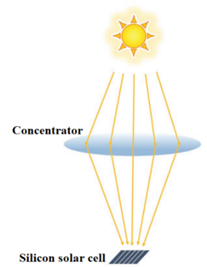Figure 1. The concentrated solar cell device studied [14, 17]

2.2 Mathematical model

As it is well known, the easiest equivalent electronic circuit of a photovoltaic solar cell is a source of current in parallel with a diode and a shunt resistance. Furthermore, the internal losses caused by the flow of current are represented by a series resistance . This electronic equivalent model is represented in Figure ‎2.

According to the theory presented by Segev et al. , adding a concentrator to a photovoltaic solar junction is represented in the equations describing the equivalent electronic circuit by multiplying the short circuit current by a factor C corresponding to the concentration ratio. Moreover, by considering the shunt resistances very big, the shunt currents are neglected. Therefore, the equations describing the behavior of a concentrated junction solar cell are shown in what follows [10, 19].

## 2.png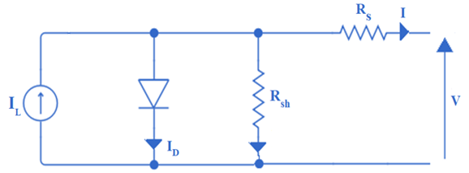Figure 2. Electronic equivalent circuit of a CPV solar cell

The power p at the output of the concentrated junction solar cell is given by the following Eq. (1):

$P=I . V$     (1)

From the electrical circuit exhibited in Figure 2, the equation of I can be found and expressed in Eq. (2):

$\mathbf{I}=\mathbf{I}_{\mathbf{L}}-\mathbf{I}_{\mathbf{D}}$      (2)

where, ID is the diode dark current which is expressed by the following :

$\mathbf{I}_{\mathrm{D}}=\mathbf{I}_{0} \cdot\left(\exp \frac{\mathbf{q}\left(\mathbf{V}+\mathbf{I R}_{s}\right)}{\mathbf{n K}_{\mathrm{B}} \mathbf{T}}-\mathbf{1}\right)$      (3)

$\mathrm{I}_{0}=\mathrm{I}_{0}\left(\mathrm{~T}_{\mathrm{r} 1}\right) \cdot\left(\frac{\mathrm{T}}{\mathrm{T}_{\mathrm{r} 1}}\right)^{\frac{3}{\mathrm{n}}} \cdot \exp \frac{-\mathbf{q V _ { \mathrm { g } } ( \mathrm { T } _ { \mathrm { r } 1 } )}}{\mathrm{nK}_{\mathrm{B}}\left(\frac{\mathbf{1}}{\mathbf{T}}-\frac{1}{\mathrm{~T}_{\mathrm{r} 1}}\right)}$      (4)

$I_{0}\left(T_{r 1}\right)=I_{s c}\left(T_{r 1}\right) \cdot\left(\exp \frac{q V_{o c}\left(T_{r 1}\right)}{n K_{B} T_{r 1}}-1\right)$     (5)

where, I0 is the saturation current of the diode, q is the charge of an electron, Rs is the series resistance, Tr1 is 25℃, n is the diode ideality factor, Vg is the band voltage, Isc is the short circuit current, and Voc is the open-circuit voltage.

The subcell photocurrent IL is described in the following Eqns. (6), (7) [10, 18]:

$\mathrm{I}_{\mathrm{L}}=\mathrm{I}_{\mathrm{L}}\left(\mathrm{T}_{\mathrm{r} 1}\right)+\mathrm{K}_{0} \cdot\left(\mathrm{T}-\mathrm{T}_{\mathrm{r} 1}\right)$     (6)

$\mathrm{I}_{\mathrm{L}}\left(\mathrm{T}_{\mathrm{r} 1}\right)=\frac{\mathrm{E} \mathrm{C}}{1000} \cdot \mathrm{I}_{\mathrm{sc}}$      (7)

where, K0 is the current-temperature coefficient from Table 1, E is the received irradiance (W/m²), and C is the concentration ratio.

The expression of the series resistance Rs is given by the following Eqns. (8), (9) :

$\mathrm{R}_{s}=-\frac{\mathrm{dV}}{\mathrm{dI}_{\mathrm{V}_{\mathrm{oc}}}}-\frac{1}{\mathrm{X}_{\mathrm{v}}}$      (8)

$\mathrm{X}_{\mathrm{V}}=\frac{\mathrm{q} \mathrm{}_{0}\left(\mathrm{~T}_{\mathrm{r} 1}\right)}{\mathrm{nK}_{\mathrm{B}} \mathrm{T}_{\mathrm{r} 1}} \cdot \exp \frac{\mathrm{qV} \mathrm{oc}\left(\mathrm{T}_{\mathrm{r} 1}\right)}{\mathrm{nK}_{\mathrm{B}} \mathrm{T}_{\mathrm{r} 1}}-\frac{1}{\mathrm{X}_{\mathrm{v}}}$      (9)

2.3 Computation program

The computation algorithm for calculating the current and the maximal power produced are presented in Figure 3. We first begin by providing the technical specifications of the silicon solar junction. Then, the mathematical model for calculating the current and the power of a concentrated photovoltaic solar cell is implemented under MATLAB environment. Newton-Raphson numerical method is used to solve the complex equations. Besides, the effect of changing the concentration ratio, the temperature of the cell, and the irradiance is studied. Finally, I-V curves and P-V curves are plotted using MATLAB, and a sensitivity study is conducted by exporting results to Excel spreadsheets.

## 3.pngFigure 3. Computation program for the study of the CPV cell

3. Results and Discussion

3.1 Parametric study

Figure 4 shows the effect of the cell's temperature on the I-V curve and the P-V curve of a concentrated photovoltaic solar cell with a concentrator of 3 as concentration ratio, and 1000 W/m² of irradiance.

It is observed from the I-V curves that while increasing the cell's temperature, the voltage decreases, and the current increases. Moreover, the P-V curves show that there is an inverse relationship between the temperature of the cell and the maximal power produced. In fact, the increase of the temperature of the cell from 20℃ to 90℃ decreases the maximal power produced from 8.89 W to 6.79 W. Therefore, adding a passive or active cooling technology to the concentrated junction solar cells should be considered while optimizing the power of such efficient solar cells.

Figure 5 shows the effect of the concentration ratio on the concentrated junction solar cell. The initial assumptions are a temperature of the cell of 30℃, and an irradiance of 1kW/m².

It is exhibited that the increase of the concentration ratio makes the current rising. Moreover, the power of the cell is empowered strongly with the increase of the concentration ratio. The maximal power produced by a concentrated junction solar cell goes from 2.95 W with a concentration ratio of 1 to 1073 W with a concentration ratio of 350. Therefore, studying in an in-depth and experimental way the best materials at the most affordable prices for the conception of the optical concentrators should be undertaken carefully during the conception of concentrated solar cells.

## 4a.png## 4b.png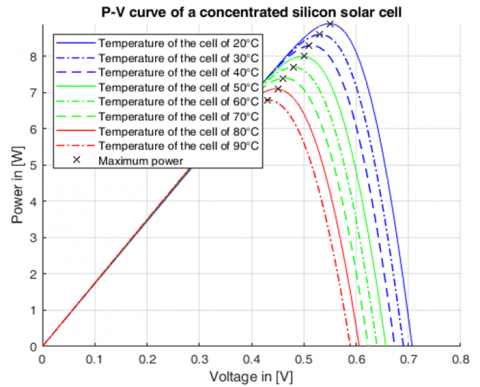Figure 4. I-V and P-V curves of the CPV cell under different cell temperatures

## 5a.png## 5b.pngFigure 5. I-V and P-V curves of the CPV cell under different concentration ratios

## 6a.png## 6b.png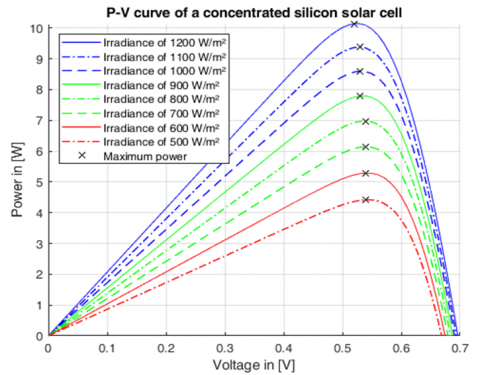Figure 6. I-V and P-V curves of the CPV cell under different irradiances

The effect of the irradiance on the voltage/current/power produced by a concentrated solar cell is shown in Figure 6. The studied concentrated solar cell has a concentration ratio of 3, and a temperature of the cell of 30°C. It is observed that the current produced rises with the increase of the irradiance. Besides, the maximal power produced increases from 4.42 W at an irradiance of 500 W/m² to 10.14 W at an irradiance of 1200 W/m². Therefore, installing the concentrated solar cells in the zones with the maximal irradiances should be studied in an in-depth way before the establishment of such devices during the engineering phase. As described in Eq. (7), there is a direct correlation between enhancing the irradiance and maximizing the current produced, and therefore, the power produced. This is why, while studying the installed CPV power plants worldwide, it is noticed that they are installed in high irradiances places in China, the USA, or South Africa .

3.2 Sensitivity study

Figure 7 emphasizes the effect of the temperature of the cell on the maximal power produced by the concentrated solar cell described in the part "Material used". One can observe that the maximal power produced by a concentrated solar cell increases linearly with the decrease of the temperature of the cell. Furthermore, a decrease of 10% of the temperature of the cell makes the maximal power increase by an average of 0.06 W.

## 7.png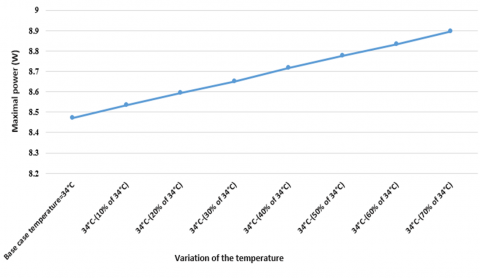Figure 7. Sensitivity of the maximal power produced by the CPV cell to the variation of the cell temperature

## 8.png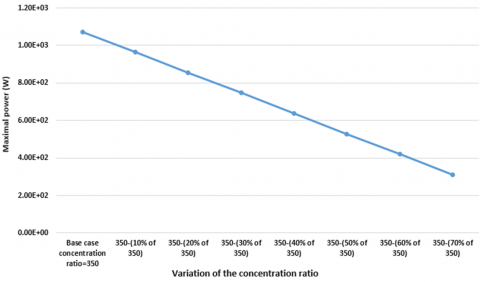Figure 8. Sensitivity of the maximal power produced by the CPV cell to the variation of the concentration ratio

Another important parameter is the concentration ratio. Figure 8 identifies the influence of the variation of the concentration ratio on the maximal power produced by a concentrated solar cell. It is evident from the figure that the maximal power is very sensitive to the concentration ratio. The maximal power decreases linearly with the decrease of the concentration ratio. Moreover, a decrease of 10% of the concentration ratio makes the maximal produced decrease by an average value of 134 W.

The last important criterion studied is the irradiance. In fact, the effect of the variation of the irradiance on the maximal power produced by a concentrated solar cell is shown in Figure 9. It is exhibited that a decrease of 10% of the irradiance makes the maximal power produced decrease by an average of 1.27 W.

## 9.png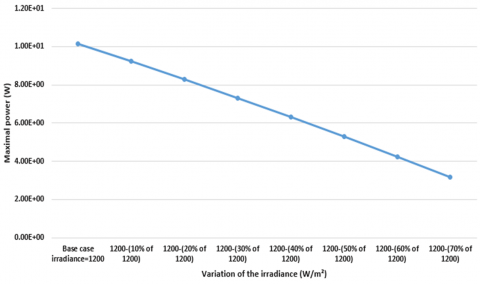Figure 9. Sensitivity of the maximal power produced by the CPV cell to the variation of the irradiance

4. Conclusions

This paper studies exclusively a low-cost concentrated photovoltaic solar cell to serve as a simulation study for the ongoing projects about experimental low-cost concentrated photovoltaic solar cells. Mathematical equations used for modeling a CPV solar cell are proposed and a computation program for the implementation of the equations under MATLAB environment is exhibited. The I-V curves and P-V curves of the CPV solar cell are plotted. The main conclusions of the study can be stated as: (a) the increase of the temperature of the CPV cell decreases both voltage and power of the cell while increases the current produced. (b) the increase of the concentration ratio or the irradiance increases the current and the power produced by a cell. (c) the most impacting parameter from the studied parameters is the concentration ratio.

References

 Bouhal, T., Aqachmar, Z., Kousksou, T., El Rhafiki, T., Jamil, A., Zeraouli, Y. (2020). Energy and economic assessment of a solar air-conditioning process for thermal comfort requirements. Solar Energy, 208: 101-14. https://doi.org/10.1016/j.solener.2020.07.045

 Aqachmar, Z., Allouhi, A., Jamil, A., Gagouch, B., Kousksou, T. (2019). Parabolic trough solar thermal power plant Noor I in Morocco. Energy, 178: 572-584. https://doi.org/10.1016/j.energy.2019.04.160

 Segev, G., Mittelman, G., Kribus, A. (2012). Equivalent circuit models for triple-junction concentrator solar cells. Solar Energy Materials and Solar Cells, 98: 57-65. https://doi.org/10.1016/j.solmat.2011.10.013

 Rezk, H., Hasaneen, E.S. (2015). A new MATLAB/Simulink model of triple-junction solar cell and MPPT based on artificial neural networks for photovoltaic energy systems. Ain Shams Engineering Journal, 6: 873-881. https://doi.org/10.1016/j.asej.2015.03.001

 Aqachmar, Z., Bouhal, T., Lahrech, K. (2020). Energetic, economic, and environmental (3 E) performances of high concentrated photovoltaic large scale installations: Focus on spatial analysis of Morocco. International Journal of Hydrogen Energy, 45(18): 10840-10861. https://doi.org/10.1016/j.ijhydene.2020.01.210

 Burhan, M., Chua, K.J.E., Ng, K.C. (2016). Sunlight to hydrogen conversion: Design optimization and energy management of concentrated photovoltaic (CPV-Hydrogen) system using micro genetic algorithm. Energy, 99: 115-128. https://doi.org/10.1016/j.energy.2016.01.048

 Das, N., Wongsodihardjo, H., Islam, S. (2015). Modeling of multi-junction photovoltaic cell using MATLAB/Simulink to improve the conversion efficiency. Renewable Energy, 74: 917-924. https://doi.org/10.1016/j.renene.2014.09.017

 Nieto-Nieto, L.M., Ferrer-Rodríguez, J.P., Muñoz-Cerón, E., Pérez-Higueras, P. (2020). Experimental set-up for testing MJ photovoltaic cells under ultra-high irradiance levels with temperature and spectrum control. Measurement, 165: 108092. https://doi.org/10.1016/j.measurement.2020.108092

 Dimroth, F., Philipps, S.P., Peharz, G., et al. (2010). Promises of advanced multi-junction solar cells for the use in CPV systems. Photovoltaic Specialists Conference (PVSC), 2010 35th IEEE, Honolulu, HI, USA, pp. 1231-1236. https://doi.org/10.1109/PVSC.2010.5614168

 González-Longatt, F.M. (2005). Model of photovoltaic module in MATLAB. Ii Cibelec 2005, pp. 1-5.

 Famoso, F., Lanzafame, R., Maenza, S., Scandura, P.F. (2015). Performance comparison between low concentration photovoltaic and fixed angle PV systems. Energy Procedia, 81: 516-525. https://doi.org/10.1016/j.egypro.2015.12.125

 Aqachmar, Z., Allouhi, A., Jamil, A. (2020). Concentrated photovoltaic (CPV) water pumping systems for agricultural uses: Sizing process, simulation and economic evaluation. International Meeting on Advanced Technologies in Energy and Electrical Engineering, vol. 2019, Hamad bin Khalifa University Press. https://doi.org/10.5339/qproc.2019.imat3e2018.7

 Luque, A.L., Andreev, V.M. (eds.) (2007). Concentrator Photovoltaics. Springer Series in Optical Sciences, vol 130. Springer, Berlin, Heidelberg. https://doi.org/10.1007/978-3-540-68798-6_11

 Sunpower. Sunpower 2003:1. https://us.sunpower.com/, accessed on August 12, 2020.

 Ohring, M. (1998). Chapter 2 - electronic devices: How they operate and are fabricated. In: Ohring MBT-R and F of EM and D, editor., San Diego: Academic Press, 37-104. https://doi.org/10.1016/B978-012524985-0/50003-9

 Sunpower solar panel 300 2009. https://us.sunpower.com/, accessed on August 12, 2020.

 Lin, Q.Q., Wang, Z.P., Snaith, H.J., Johnston, M.B., Herz, L.M. (2018). Hybrid perovskites: prospects for concentrator solar cells. Advanced Science, 5(4): 1700792. https://doi.org/10.1002/advs.201700792

 Segev, G., Kribus, A. (2013). Performance of CPV modules based on vertical multi-junction cells under non-uniform illumination. Solar Energy, 88: 120-128. https://doi.org/10.1016/j.solener.2012.11.020

 Luque, A., Hegedus, S. (2011). Handbook of Photovoltaic Science and Engineering. John Wiley & Sons. https://doi.org/10.1002/9780470974704

 Aqachmar, Z., Ben Sassi, H., Lahrech, K., Barhdadi, A. (2021). Solar technologies for electricity production: An updated review. International Journal of Hydrogen Energy, 46(60): 30790-30817. https://doi.org/10.1016/j.ijhydene.2021.06.190